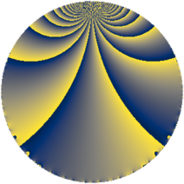# Properties

 Label 135.2.jLevel $135$ Weight $2$ Character orbit 135.j Rep. character $\chi_{135}(19,\cdot)$ Character field $\Q(\zeta_{6})$ Dimension $8$ Newform subspaces $1$ Sturm bound $36$ Trace bound $0$

# Related objects

## Defining parameters

 Level: $$N$$ $$=$$ $$135 = 3^{3} \cdot 5$$ Weight: $$k$$ $$=$$ $$2$$ Character orbit: $$[\chi]$$ $$=$$ 135.j (of order $$6$$ and degree $$2$$) Character conductor: $$\operatorname{cond}(\chi)$$ $$=$$ $$45$$ Character field: $$\Q(\zeta_{6})$$ Newform subspaces: $$1$$ Sturm bound: $$36$$ Trace bound: $$0$$

## Dimensions

The following table gives the dimensions of various subspaces of $$M_{2}(135, [\chi])$$.

Total New Old
Modular forms 48 16 32
Cusp forms 24 8 16
Eisenstein series 24 8 16

## Trace form

 $$8 q + O(q^{10})$$ $$8 q - 8 q^{10} + 12 q^{11} + 4 q^{16} - 8 q^{19} - 12 q^{20} - 4 q^{25} - 24 q^{26} - 12 q^{29} + 4 q^{31} - 4 q^{34} + 24 q^{35} - 4 q^{40} + 24 q^{41} + 24 q^{44} - 16 q^{46} - 4 q^{49} - 24 q^{50} + 24 q^{55} + 12 q^{56} - 24 q^{59} - 8 q^{61} + 32 q^{64} + 12 q^{70} - 72 q^{71} + 12 q^{74} - 12 q^{76} + 16 q^{79} + 48 q^{80} + 16 q^{85} + 36 q^{86} + 24 q^{89} - 24 q^{91} + 20 q^{94} - 12 q^{95} + O(q^{100})$$

## Decomposition of $$S_{2}^{\mathrm{new}}(135, [\chi])$$ into newform subspaces

Label Dim $A$ Field CM Traces $q$-expansion
$a_{2}$ $a_{3}$ $a_{5}$ $a_{7}$
135.2.j.a $8$ $1.078$ $$\Q(\zeta_{24})$$ None $$0$$ $$0$$ $$0$$ $$0$$ $$q+\zeta_{24}^{6}q^{2}-\zeta_{24}^{3}q^{4}+(\zeta_{24}-\zeta_{24}^{3}+\cdots)q^{5}+\cdots$$

## Decomposition of $$S_{2}^{\mathrm{old}}(135, [\chi])$$ into lower level spaces

$$S_{2}^{\mathrm{old}}(135, [\chi]) \cong$$ $$S_{2}^{\mathrm{new}}(45, [\chi])$$$$^{\oplus 2}$$